# MCAT Physical : Other Harmonic Motion Principles

## Example Questions

### Example Question #1 : Other Harmonic Motion Principles

A long pendulum with a length of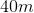has a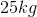mass attached to the end of it. Approximately how long does it take for the pendulum to swing one time from its maximum displacement?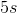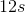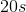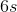Explanation:

We are asked for the period of the pendulum, or the time it takes to make one full revolution or swing.

The period of a pendulum is given by this formula: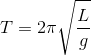Where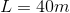and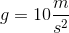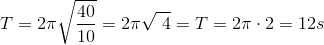It takes roughly 12 seconds for the pendulum to make a full swing. Note that the mass attached to the end of the pendulum is irrelevant.

### Example Question #2 : Other Harmonic Motion Principles

A spring is compressed as far possible and is not permitted to expand. What can be said about its potential energy and its kinetic energy?

Its potential energy is at a maximum and its kinetic energy is at a minimum

Its potential energy is at a minimum and its kinetic energy is at a maximum

The total energy of the spring is zero

Its potential and kinetic energy are both at a maximum

Its potential energy and kinetic energy are both at a minimum

Its potential energy is at a maximum and its kinetic energy is at a minimum

Explanation:

In this case, the kinetic energy of the spring is at a minimum. This is because, as the question indicates, the spring is not moving. At the same time, because the spring is compressed as far is it can be compressed, we know that its potential energy is at a maximum. The total energy of the spring therefore cannot be zero.

### Example Question #3 : Other Harmonic Motion Principles

Which of the following changes to a pendulum would decrease its period?

Increasing the length of the pendulum

Starting the pendulum from a greater height

Increasing the mass of the weight at the end of the pendulum

Decreasing the gravitational attraction involved

Decreasing the density of the pendulum